### What Is Python Matplotlib?¶

matplotlib.pyplot is a plotting library used for 2D graphics in python programming language. It can be used in python scripts, shell, web application servers, and other graphical user interface toolkits.

There are several toolkits that are available that extend python matplotlib functionality. Some of them are separate downloads, others can be shipped with the matplotlib source code but have external dependencies.

• Basemap: It is a map plotting toolkit with various map projections, coastlines and political boundaries.
• Cartopy: It is a mapping library featuring object-oriented map projection definitions, and arbitrary point, line, polygon and image transformation capabilities.
• Excel tools: Matplotlib provides utilities for exchanging data with Microsoft Excel.
• Mplot3d: It is used for 3-D plots.
• Natgrid: It is an interface to the natgrid library for irregular gridding of the spaced data.

What will you learn here:

1) Introductory These tutorials cover the basics of creating visualizations with Matplotlib, as well as some best practices in using the package effectively.

2) Intermediate These tutorials cover some of the more complicated classes and functions in Matplotlib. They can be useful for particular custom and complex visualizations.

3) Advanced These tutorials cover advanced topics for experienced Matplotlib users and developers.

4) Colors Matplotlib has support for visualizing information with a wide array of colors and colormaps. These tutorials cover the basics of how these colormaps look, how you can create your own, and how you can customize colormaps for your use case.

5) Text matplotlib has extensive text support, including support for mathematical expressions, truetype support for raster and vector outputs, newline separated text with arbitrary rotations and Unicode support. These tutorials cover the basics of working with text in Matplotlib.

6) Toolkits These tutorials cover toolkits designed to extend the functionality of Matplotlib in order to accomplish specific goals.

### Python Matplotlib : Types of Plots¶

There are various plots which can be created using python matplotlib. Some of them are listed below:

• Bar Graph
• Histogram
• Scatter Plot
• Area Plot
• Pie Plot

### Matplotlib, pyplot and pylab: What is the relation between them?¶

Matplotlib is the whole package; matplotlib.pyplot is a module in matplotlib; and pylab is a module that gets installed alongside matplotlib.

Pyplot provides the state-machine interface to the underlying object-oriented plotting library. The state-machine implicitly and automatically creates figures and axes to achieve the desired plot. For example:

In :
# sphinx_gallery_thumbnail_number = 3
import matplotlib.pyplot as plt
import numpy as np

x = np.linspace(0, 2, 100)

plt.plot(x, x, label='linear')
plt.plot(x, x**3, label='cubic')

plt.xlabel('x label')
plt.ylabel('y label')

plt.title("Simple Plot")

plt.legend()

plt.show()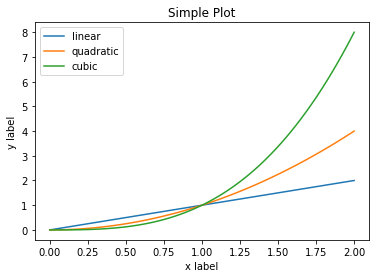### Working with multiple figures and axes¶

MATLAB, and pyplot, have the concept of the current figure and the current axes. All plotting commands apply to the current axes. The function gca() returns the current axes (a matplotlib.axes.Axes instance), and gcf() returns the current figure. Normally, you don’t have to worry about this, because it is all taken care of behind the scenes. Below is a script to create two subplots.

In :
import numpy as np
import matplotlib.pyplot as plt

def f(t):
return np.exp(-t) * np.cos(2*np.pi*t)

t1 = np.arange(0.0, 5.0, 0.1)
t2 = np.arange(0.0, 5.0, 0.02)

plt.figure(1)
plt.subplot(211)
plt.plot(t1, f(t1), 'bo', t2, f(t2), 'k')

plt.subplot(212)
plt.plot(t2, np.cos(2*np.pi*t2), 'r--')
plt.show()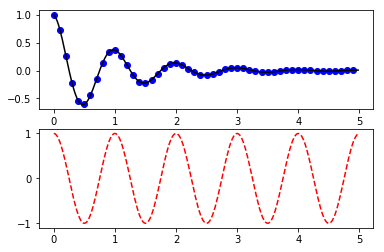In :
import matplotlib.pyplot as plt
plt.figure(1)                # the first figure
plt.subplot(211)             # the first subplot in the first figure
plt.plot([1, 2, 3])
plt.subplot(212)             # the second subplot in the first figure
plt.plot([4, 5, 6])

plt.figure(2)                # a second figure
plt.plot([4, 5, 6])          # creates a subplot(111) by default

plt.figure(1)                # figure 1 current; subplot(212) still current
plt.subplot(211)             # make subplot(211) in figure1 current
plt.title('Easy as 1, 2, 3') # subplot 211 title

Out:
<matplotlib.text.Text at 0x7f9a61e24450>

### Working with text¶

The text() command can be used to add text in an arbitrary location, and the xlabel(), ylabel() and title() are used to add text in the indicated locations (see Text introduction for a more detailed example)

In :
import numpy as np
import matplotlib.pyplot as plt

# Fixing random state for reproducibility
np.random.seed(19680801)

mu, sigma = 100, 15
x = mu + sigma * np.random.randn(10000)

# the histogram of the data
n, bins, patches = plt.hist(x, 50, normed=1, facecolor='g', alpha=0.75)

plt.xlabel('Smarts')
plt.ylabel('Probability')
plt.title('Histogram of IQ')
plt.text(60, .025, r'$\mu=100,\ \sigma=15$')
plt.axis([40, 160, 0, 0.03])
plt.grid(True)
plt.show()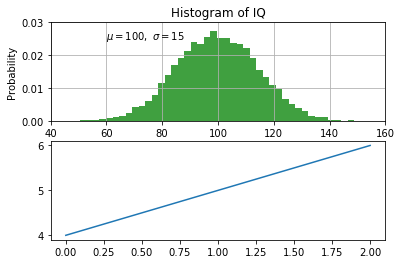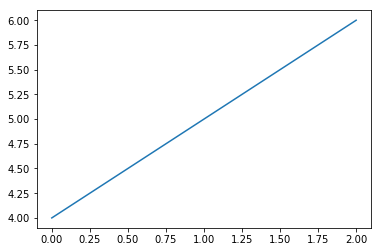All of the text() commands return a matplotlib.text.Text instance. Just as with lines above, you can customize the properties by passing keyword arguments into the text functions or using setp():

In :
import numpy as np
import matplotlib.pyplot as plt

# Fixing random state for reproducibility
np.random.seed(19680801)

mu, sigma = 100, 15
x = mu + sigma * np.random.randn(10000)

# the histogram of the data
n, bins, patches = plt.hist(x, 50, normed=1, facecolor='g', alpha=0.75)

plt.xlabel('Smarts')
plt.ylabel('Probability')
plt.title('Histogram of IQ')
t = plt.xlabel('my data', fontsize=14, color='red')
plt.text(60, .025, r'$\mu=100,\ \sigma=15$')
plt.axis([40, 160, 0, 0.03])
plt.grid(True)
plt.show()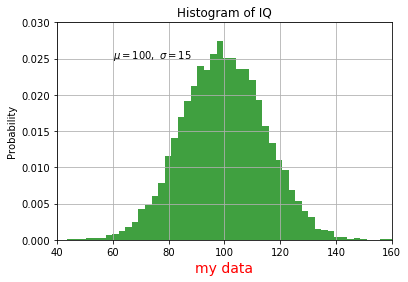### Using Mathematical Expressions In Text¶

matplotlib accepts TeX equation expressions in any text expression. For example, to write the expression in the title, you can write a TeX expression surrounded by dollar signs:

In :
import numpy as np
import matplotlib.pyplot as plt

# Fixing random state for reproducibility
np.random.seed(19680801)

mu, sigma = 100, 15
x = mu + sigma * np.random.randn(10000)

# the histogram of the data
n, bins, patches = plt.hist(x, 50, normed=1, facecolor='g', alpha=0.75)

plt.xlabel('Smarts')
plt.ylabel('Probability')
plt.title('Histogram of IQ')
plt.title(r'$\sigma_i=15$')
t = plt.xlabel('my data', fontsize=14, color='red')
plt.text(60, .025, r'$\mu=100,\ \sigma=15$')
plt.axis([40, 160, 0, 0.03])
plt.grid(True)
plt.show()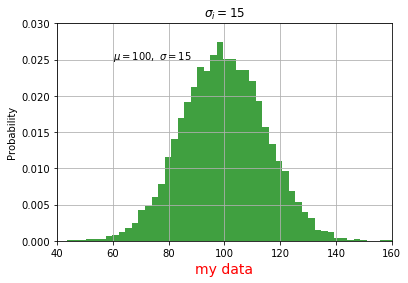The r preceding the title string is important – it signifies that the string is a raw string and not to treat backslashes as python escapes. matplotlib has a built-in TeX expression parser and layout engine and ships its own math fonts. Thus you can use mathematical text across platforms without requiring a TeX installation. For those who have LaTeX and dvipng installed, you can also use LaTeX to format your text and incorporate the output directly into your display figures or saved postscript.

### Annotating text¶

The uses of the basic text() command above place text at an arbitrary position on the Axes. A common use for text is to annotate some features of the plot, and the annotate() method provides helper functions to make annotations easy. In an annotation, there are two points to consider: the location being annotated represented by the argument xy and the location of the text xytext. Both of these arguments are (x,y) tuples.

In :
import numpy as np
import matplotlib.pyplot as plt

ax = plt.subplot(111)

t = np.arange(0.0, 5.0, 0.01)
s = np.cos(2*np.pi*t)
line, = plt.plot(t, s, lw=2)

plt.annotate('local max', xy=(2, 1), xytext=(3, 1.5),
arrowprops=dict(facecolor='black', shrink=0.05),
)

plt.ylim(-2,2)
plt.show()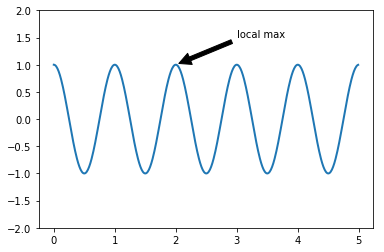### Logarithmic And Other Non-linear Axes¶

matplotlib.pyplot supports not only linear axis scales but also logarithmic and logit scales. This is commonly used if data spans many orders of magnitude. Changing the scale of an axis is easy:

plt.xscale(‘log’)

An example of four plots with the same data and different scales for the y-axis is shown below.

In :
import numpy as np
import matplotlib.pyplot as plt

from matplotlib.ticker import NullFormatter  # useful for logit scale

# Fixing random state for reproducibility
np.random.seed(19680801)

# make up some data in the interval ]0, 1[
y = np.random.normal(loc=0.5, scale=0.4, size=1000)
y = y[(y > 0) & (y < 1)]
y.sort()
x = np.arange(len(y))

# plot with various axes scales
plt.figure(1)

# linear
plt.subplot(221)
plt.plot(x, y)
plt.yscale('linear')
plt.title('linear')
plt.grid(True)

# log
plt.subplot(222)
plt.plot(x, y)
plt.yscale('log')
plt.title('log')
plt.grid(True)

# symmetric log
plt.subplot(223)
plt.plot(x, y - y.mean())
plt.yscale('symlog', linthreshy=0.01)
plt.title('symlog')
plt.grid(True)

# logit
plt.subplot(224)
plt.plot(x, y)
plt.yscale('logit')
plt.title('logit')
plt.grid(True)
# Format the minor tick labels of the y-axis into empty strings with
# NullFormatter, to avoid cumbering the axis with too many labels.
plt.gca().yaxis.set_minor_formatter(NullFormatter())
# Adjust the subplot layout, because the logit one may take more space
# than usual, due to y-tick labels like "1 - 10^{-3}"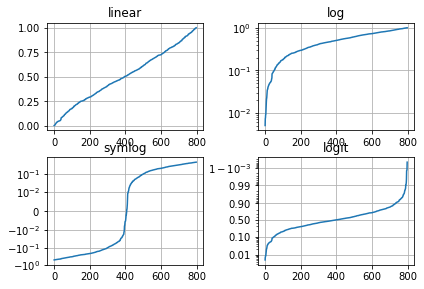Dolly Solanki